# Intermediate Geometry : How to find if rhombuses are similar

## Example Questions

### Example Question #1 : How To Find If Rhombuses Are Similar

Given: Rhombusesand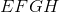.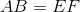True, false, or undetermined: Rhombus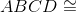Rhombus.

True

Undetermined

False

Undetermined

Explanation:

Two figures are congruent by definition if all of their corresponding sides are congruent and all of their corresponding angles are congruent.

By definition, a rhombus has four sides of equal length.  If we let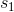be the common sidelength of Rhombusand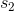be the common sidelength of Rhombus, then, since, it follows that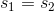, so corresponding sides are congruent. However, no information is given about their angle measures. Therefore, it cannot be determined whether or not the two rhombuses are congruent.

### Example Question #2 : How To Find If Rhombuses Are Similar

Given: Rhombusesand.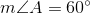and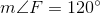True, false, or undetermined: Rhombus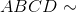Rhombus.

False

Undetermined

True

True

Explanation:

Two figures are similar by definition if all of their corresponding sides are proportional and all of their corresponding angles are congruent.

By definition, a rhombus has four sides that are congruent. If we letbe the common sidelength of Rhombusandbe the common sidelength of Rhombus, it can easily be seen that the ratio of the length of each side of the former to that of the latter is the same ratio, namely,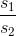.

Also, a rhombus being a parallelogram, its opposite angles are congruent, and its consecutive angles are supplementary. Therefore, since, it follows that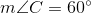, and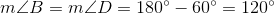. By a similar argument,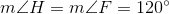and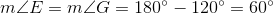. Therefore,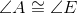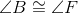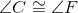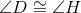Since all corresponding sides are proportional and all corresponding angles are congruent, it holds that RhombusRhombus.